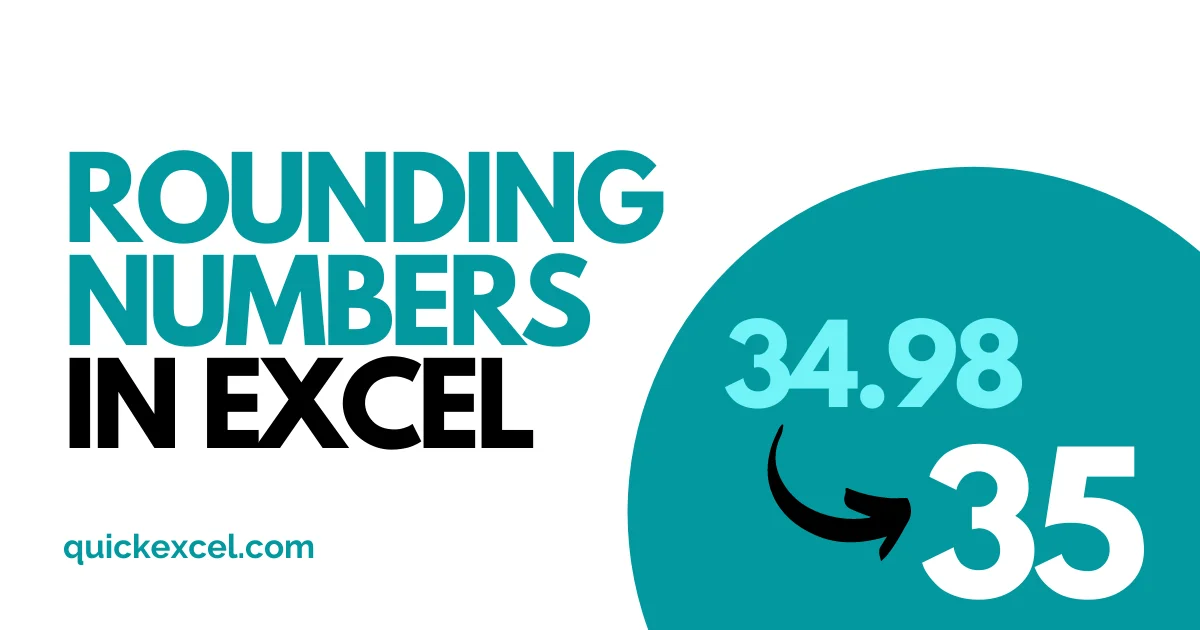# [Step-by-Step] How to Round Numbers in Excel?In this tutorial, we will learn to round numbers in multiple ways in Microsoft Excel. We can round numbers either via the Home tab or by using different formulas like the ROUND, ROUNDUP, and ROUNDDOWN functions in Excel.

Also read: How to Stop Excel from Rounding?

## Steps to round numbers in Excel

To directly round numbers without using a formula, here’s what you can do.

• Type a decimal number in a cell with more than 2 or 3 digits after the decimal point.
• Go to the Home tab.
• Under the Number section, select the Increase Decimal or Decrease Decimal icon to round up that number.

You can see that the number has been rounded up after selecting the Decrease Decimal option in Excel.

## Using the ROUND function in Excel

The ROUND function is created to round numbers in Excel. Let’s see how we can round numbers with this function.

• Type a decimal number in a cell.
• Type =ROUND( in another blank cell.
• Select the decimal number.
• Put a comma and type the number of digits you want to have after the decimal point.
• Close brackets and press ENTER.

You can see that the ROUND formula has rounded up the number in the cell. Let us try another example by putting 0 digits to be shown.

You can see that the ROUND function has rounded up the two numbers into whole numbers.

Note – Any decimal number that has a number equal to or greater than 0.5 after decimals, then that number gets rounded up to the nearest highest whole number if 0 digits have been applied in the ROUND function.

## Using the ROUNDUP function in Excel

The ROUNDUP function is used to round up decimal numbers to the nearest highest decimal point in Excel. Let’s see how we can round up numbers with this function.

• Type a decimal number in a cell.
• Type =ROUNDUP( in another blank cell.
• Select the decimal number.
• Put a comma and type the number of digits you want to have after the decimal point.
• Close brackets and press ENTER.

We can see that the two numbers have been rounded up by the ROUNDUP function in Excel to the nearest highest decimal point.

Let’s now see what results do we get after applying 0 digits to be seen after the decimal point

You can see that both numbers irrespective of any number after the decimal point, get rounded up to the nearest highest whole number by the ROUNDUP function.

You can clearly figure out the difference between the ROUND and the ROUNDUP function when it comes to applying 0 digits in the num_digits argument. The ROUND function will not round up to the nearest whole number until any number equal to greater than 0.5 is entered after the decimal point.

## Using the ROUNDDOWN function in Excel

The ROUNDDOWN function, as the name suggests, is contrary to the ROUNDUP function in Excel. It is used to round down decimal numbers to the nearest lowest decimal point. Let’s see how we can round down numbers with this function.

• Type a decimal number in a cell.
• Type =ROUNDDOWN( in another blank cell.
• Select the decimal number.
• Put a comma and type the number of digits you want to have after the decimal point.
• Close brackets and press ENTER.

You can see that the ROUNDDOWN function has rounded down the two numbers in Excel. There is little to no difference in the numbers except that there are only 2 digits to be seen after the decimal point now.

Let’s see the result after putting 0 digits in the num_digits argument.